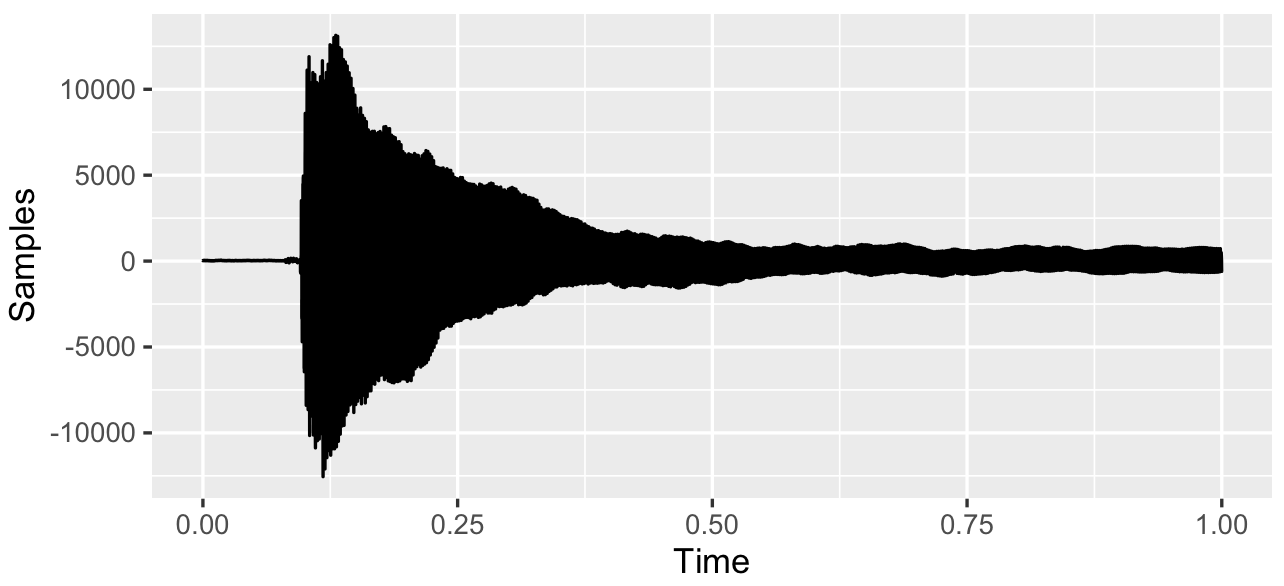First of my experiments on audio modelling using gaussian processes. Here, I construct a GP that, when sampled, plays middle c the way a grand piano would.

I tried using the Spectral Mixture kernel and the Gaussian Process Convolution Model for this, but neither seemed to work well for me (numerical issues with the covariance, likelihood has too many modes respectively). However, I did learn about the vec trick and that sympy handles infinite precision - for more info check out my scicomp question here.

Due to the difficulty in automating the inference for the kernel, I decided to try my hand at writing it out myself. It’s a simple signal, so it was pretty easy.

The signal:Its sample autocorrelation by time:Key observations about the signal are:

1. It’s highly periodic, with the same frequency.
2. The autocorrelation decays to zero if you go far enough away.
3. The signal is heteroskedastic, with the variance decreasing over time.
4. The autocorrelation function seems to change at some point.

I designed a kernel with these charecteristics. It may’ve been a total accident (haven’t checked yet), but this works! The result:

Code (it’s a bit messy):

R Code

## 2020

### Efficient Gaussian Process Computation

I’ll try to give examples of efficient gaussian process computation here, like the vec trick (Kronecker product trick), efficient toeliptz and circulant matrix computations, RTS smoothing and Kalman filtering using state space representations, and so on.

### Gaussian Process Speech Synthesis (Draft)

Very untidy first working draft of the idea mentioned on the efficient computation page. Here, I fit a spectral mixture to some audio data to build a “generative model” for audio. I’ll implement efficient sampling later, and I’ll replace the arbitrary way this is trained with an LSTM-RNN to go straight from text/spectrograms to waveforms.

# Random Projects

### Gaussian Process Middle C

First of my experiments on audio modelling using gaussian processes. Here, I construct a GP that, when sampled, plays middle c the way a grand piano would.

Consider: